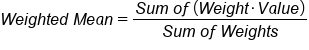+
Weighted Mean
Central Tendency, Variation, and Distributions
0
of 90 possible points
Common Core: 6.SP.5

# Weighted Mean

Author: Sophia Tutorial
##### Description:

Calculate a weighted mean for a given scenario.

(more)

Sophia’s self-paced online courses are a great way to save time and money as you earn credits eligible for transfer to many different colleges and universities.*

No credit card required

46 Sophia partners guarantee credit transfer.

299 Institutions have accepted or given pre-approval for credit transfer.

* The American Council on Education's College Credit Recommendation Service (ACE Credit®) has evaluated and recommended college credit for 33 of Sophia’s online courses. Many different colleges and universities consider ACE CREDIT recommendations in determining the applicability to their course and degree programs.

Tutorial
what's covered
This tutorial will explain finding the weighted mean of a data set. Our discussion breaks down as follows:

1. Calculating Weighted Mean
1. Example 1
2. Example 2

## 1. Calculating Weighted Mean

Sometimes, all of the values in your data set will not be weighted the same. If you are calculating the mean of a data set where there are weighted values, you will need to account for these differences by using a weighted mean/average.

When calculating the weighted mean, it is important to keep the following formula in mind:

formula
Weighted MeanSometimes in an academic course, exams are weighted for a certain percentage of the grade, and the final is weighted for a greater percentage.

term to know

Weighted Mean/Average
A way of calculating a mean when not all the values count for the same amount. Each value should be multiplied by its weight and added together, then divide the sum by the sum of the weights.

1a. Example 1

Suppose a statistics course has four tests which comprise the course grade, but the final exam is weighted three times as much as the others.

A student receives the following scores:

• Exam 1: 78
• Exam 2: 83
• Exam 3: 82
• Final: 94

What is this student's grade for the course? How do you find the mean?

You would first multiply each of the tests by their weights. Count each of the first three tests as each one test. However, count the final exam as three tests because it’s weighted three times as much:This weighted average, or the weighted mean, is 87.5.

big idea
It's important to note that you are counting these not as four tests, but essentially more like six tests, because the final one counts for three of the others. Therefore, you divide by 6 in the end.

1b. Example 2
Suppose a student named Sam is taking a class where each of the grades has different importance. Participation is worth 10% of Sam's grade, homework is worth 25%, quizzes are worth 50%, and tests are worth 15%. This is indicated with the following table:

Assignment Weight
Participation 10%
Homework 25%
Quizzes 50%
Tests 15%

In this class, Sam earned the following scores:

Assignment Score
Participation 100
Homework 50
Quizzes 70
Tests 93

To calculate the weighted mean, we need to multiply each score that she received by the corresponding weight, add the values together, and divide by the total weight.The weighted mean is 71.45, which tells us that Sam received a final grade of 71.45.

summary
The mean, or what is meant by the term "average," is one measure of center that we can use. Weighted averages can be found by multiplying each value times its weight and counting it, essentially, that many times.

Good luck!

Source: Adapted from Sophia tutorial by Jonathan Osters.

Terms to Know
Mean

The "average" value of a data set. It is obtained by dividing the sum of the values by the number of values in the set.

Summation Notation

A notation that uses the Greek letter sigma to state that values should be added together.

Weighted Mean/Average

A way of calculating a mean when not all the values count for the same amount. Each value should be multiplied by its weight and added together, then divide the sum by the sum of the weights.

Formulas to Know
MeanWeighted MeanRating